# KVPY-SA 2018 Physics Paper with Solutions

The Kishore Vaigyanik Protsahan Yojana (KVPY) is a national level examination conducted by the Department of Science and Technology. The objective of the program is to motivate students to pursue a career in science research. The test is conducted at three different levels namely SA, SX and SB. The applicants are advised to practise previous years’ question papers in order to clear the aptitude test. KVPY- SA 2018 Physics paper is answered on this page. PDF download of the question paper is also available.

### KVPY SA 2018 - Physics

Question 1: A block of wood is floating on water at 0°C with volume V0 above water. When the temperature of water increases from 0 to 10°C, the change in the volume of the block that is above water is best described schematically by the graph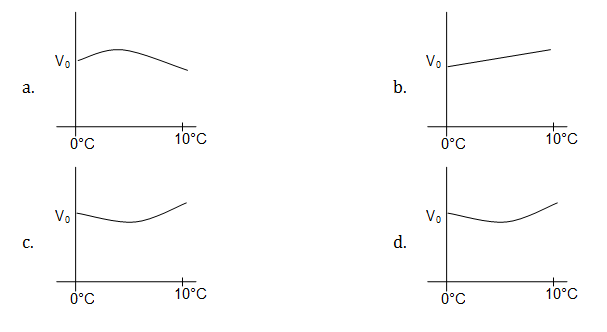Solution:

Change in volume can occur due to following reason:

(i) Density/volume of fluid changes due to increase or decrease in temperature.

(ii) The volume of material which is immersed changes due to temperature change [This argument need not to be considered as change in volume of the material is infinitesimal as co-efficient of volume thermal expansion has a very low value]

• For floating condition we can write [T = O°C] [Archimedes – principle]

Weight of the block = Buoyant force on the block

W = FB

W = ρwatergVimmersed ……(1)

• As buoyant force depend on the density of water if it changes due to temperature change [ρwater is maximum at 4°C]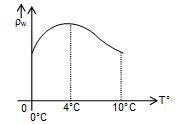From (1)

mg =ρwaterg Vimmersed [m = mass of block]

Vimmersed= m/ρwater

Volume outside water:-

Vo = Vtotal – Vimmersed

0 – 4°C Density ↑ Vimmersed ↓ Vo

4°C – 10°C Density ↓ Vimmersed ↑ Vo

Hence volume outside Block changes according to immersed volume which depend on density of water.

Above statement written to understand that density variation curve & volume outside water of the block with temperature change is same.

$V_{0}\propto \frac{1}{V_{i}}$

$V_{i}\propto \frac{1}{\rho _{water}}$

$V_{0}\propto \rho _{water}$

Question 2: A very large block of ice of the size of a volleyball court and of uniform thickness of 8m is floating on water. A person standing near its edge wishes to fetch a bucketful of water using a rope. The smallest length of rope required for this is about

1. a. 3.6 m
2. b. 1.8 m
3. c. 0.9 m
4. d. 0.4 m

Solution:

From Archimedes principle:-

Weight of ice-Block = Buoyant force on block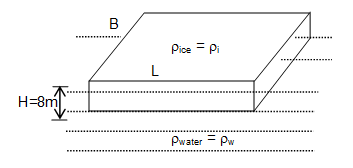W = FB

ρi. gV = ρw g Vimmersed

$V_{immersed}\left [ V_{i} \right ]=\left [ \frac{\rho _{i}}{\rho _{w}} \right ]\times V$

$LBH_{immersed}=\left [ \frac{\rho _{i}}{\rho _{w}} \right ]\times LBH$

$H_{immersed}=\left [ \frac{\rho _{i}}{\rho _{w}} \right ]\times H$

We know that

Density of ice, ρi = 0.9 gm/cm3

Density of water

ρw = 1 gm/cm3

Hi = 0.9H

Hi = 7.2 m

So Height outside water:

Ho=8 – 7.2

Ho = 0.8m

Nearest value is 0.9m

Hence, the smallest length of rope required is 0.9m.

Question 3: A box filled with water has a small hole on its side near the bottom. It is dropped from the top of a tower. As it falls, a camera attached on the side of the box records the shape of the water stream coming out of the hole. The resulting video will show

1. a. the water coming down forming a parabolic stream
2. b. the water going up forming a parabolic stream
3. c. the water coming out in a straight line
4. d. no water coming out

Solution:

When a filled box with water with small hole falls freely, then pressure is same at inside and outside of hole i.e. atmospheric pressure. No water will come out of hole as there is no pressure difference.

Option (d) is correct

Question 4: An earthen pitcher used in summer cools water in it essentially by evaporation of water from its porous surface. If a pitcher carries 4 kg of water and the rate of evaporation is 20 g per hour, temperature of water in it decreases by ΔT in two hours. The value of ΔT is close to (ratio of latent of evaporation to specific heat of water is 540 °C)

1. a. 2.7 °C
2. b. 4.2 °C
3. c. 5.4 °C
4. d. 10.8°C

Solution:

As evaporation occur the temperature of the water decreases as it is a cooling process

Rate of evaporation of water = 20 g per hour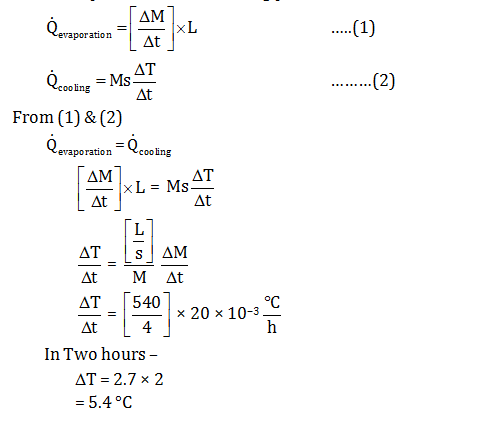Question 5: Two plane mirrors are kept on a horizontal table making an angle θ with each other as shown schematically in the figure. The angle θ is such that any ray of light reflected after striking both the mirrors return parallel to its incident path. For this to happen, the value of θ should be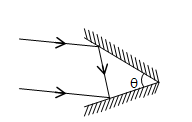1. a. 30°
2. b. 45°
3. c. 60°
4. d. 90°

Solution: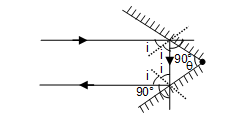Angle of deviation [δ]:-

δ = 360°– 2θ

given:- δ = 180°

180° = 360° – 2θ

θ = 90°

Hence; option (d) is correct

Question 6: A certain liquid has a melting point of –50°C and a boiling point of 150°C. A thermometer is designed with this liquid and it’s melting and boiling points are designated as 0° L and 100°L. The melting and boiling points of water on this scale are

1. a. 25° L and 75° L, respectively
2. b. 0° L and 100° L, respectively
3. c. 20° L and 70° L, respectively
4. d. 30° L and 80° L, respectively

Solution:

This question is based on calibration of thermometer on various temperature scale in which the value of two standard reference point are given i.e. melting and boiling points.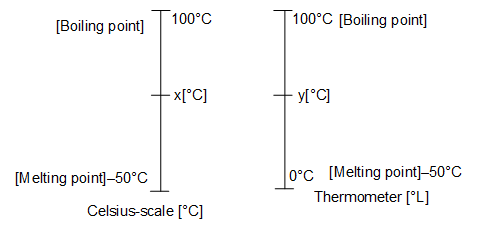$\frac{x-[-50]}{150-[-50]}=\frac{y-0}{100-0}$

$\frac{x+50}{200}=\frac{y}{100}$

$x^{0}C+50=2y^{0}L$

Now for water:-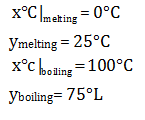Alternate solution:-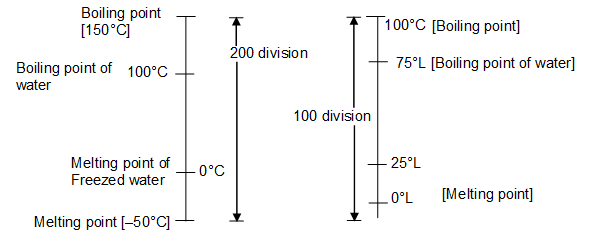On Comparison

1°L = 2°C

Question 7: One can define an alpha–Volt (α V) to be the energy acquired by an α particle when it is accelerated by a potential of 1 Volt. For this problem you may take a proton to 2000 times heavier than an electron. Then

1. a. 1 ∝ V = 1 eV/4000
2. b. 1 ∝ V = 2eV
3. c. 1 ∝ V = 8000 eV
4. d. 1 ∝ V = 1 eV

Solution:

From work kinetic - energy theorem:

Work done= Change in kinetic energy

W = ΔK

Work done =q [ΔV]

q = charge

ΔV = potential difference

qα = 2qe

For same –potential:

ΔKα = 2ΔKe

1αV = 2eV

Hence, option (B) is correct

Question 8: In a particle accelerator, a current of 500 μA is carried by a proton beam in which each proton has a speed of 3 × 107 m/s. The cross sectional area of the beam is 1.50 mm2. The charge density in this beam in coulomb/m3 is close to–

1. a. 10–8
2. b. 10–7
3. c. 10–6
4. d. 10–5

Solution:

From current Electricity:-

i = neAVd

n = no. of electrons per unit volume

A = cross-sectional area

Vd = Drift speed

Charge-density = q/V

q/V= ne = charge on particle per unit volume [charge density]

ne = i/AVd

$=\frac{500\times 10^{-6}}{1.5\times 10^{-6}\times 3\times 10^{7}}$

= 10–5 c/m3

Hence option (D) is correct.

Question 9: Which of the following is NOT true about the total lunar eclipse?

1. a. A lunar eclipse can occur on a new moon and full moon day.
2. b. The lunar eclipse would occur roughly every month if the orbits of earth and moon were perfectly coplanar
3. c. The moon appears red during the eclipse because the blue light is absorbed in earth’s atmosphere and red is transmitted
4. d. A lunar eclipse can occur only on a full moon day

Solution:

A lunar eclipse can occur only on full moon day.

Hence option (a) is not correct and this is the required answer.

Question 10: Many exoplanets have been discovered by the transit method, wherein one monitors a dip in the intensity of the parent star as the exoplanet moves in front of it. The exoplanet has a radius R and the parent star has radius 100R. If I0 is the intensity observed on earth due to the parent star, then as the exoplanet transits

1. a. the minimum observed intensity of the parent star is 0.9 I0
2. b. the minimum observed intensity of the parent star is 0.99 I0
3. c. the minimum observed intensity of the parent star is 0.999 I0
4. d. the minimum observed intensity of the parent star is 0.9999 I0

Solution: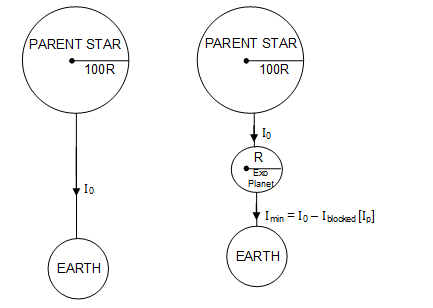Ap = effective area of exoplanet

As = effective Area of star

$\frac{I_{0}}{I_{p}}=\frac{A_{s}}{A_{p}}$

$I_{p}=\left [ \frac{A_{s}}{A_{p}} \right ]\times I_{0}$

$I=I_{0}-\left [ \frac{A_{s}}{A_{p}} \right ]\times I_{0}$

$I=I_{0}\left [ 1-\frac{R^{2}}{(100R)^{2}} \right ]$

$I=\frac{9999I_{0}}{10000}$

I = 0.9999I0

Question 11: A steady current I is set up in a wire whose cross-sectional area decreases in the direction of the flow of the current. Then, as we examine the narrowing region

1. a. the current density decreases in value
2. b. the magnitude of the electric field increases
3. c. the current density remains constant
4. d. the average speed of the moving charges remains constant

Solution:

Given :-

I = constant

Current Density $\left [ \vec{J} \right ]=\frac{I}{A}$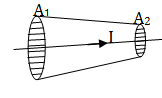$J\propto \frac{1}{A}$

Hence option (A) & (C) are wrong due to above expression

Now

$\vec{J}=\sigma \vec{E}$

$\sigma$ = conductivity of the material

$E\propto J\propto \frac{1}{A}$

magnitude of Electric-Field increases. [Option B is correct]

I = neAVd

$V_{d}\propto \frac{1}{A}$

Hence Drift speed/average speed increases along the length.

Option (D) is incorrect

Question 12: Select the correct statement about rainbow

1. a. We can see a rainbow in the western sky in the late afternoon
2. b. The double rainbow has red on the inside and violet on the outside
3. c. A rainbow has an arc shape since the earth is round
4. d. A rainbow on the moon is violet on the inside and red on the outside

Solution: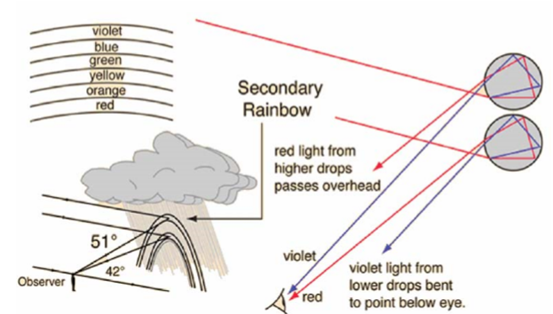Question 13: Remote sensing satellites move in an orbit that is at an average height of about 500 km from the surface of the earth. The camera onboard one such satellite has a screen on area A on which the images captured by it are formed. If the focal length of the camera lens is 50 cm, then the terrestrial area that can be observed from the satellite is close to

1. a. 2 × 103A
2. b. 106A
3. c. 1012A
4. d. 4 × 1012A

Solution:

Area of Image = m2 × area of object

Ai = m2Ao

m = magnification = Height of image/Height of object

= $=\frac{v^{2}}{u^{2}}A_{0}$

$A_{0}=A_{i}.\frac{u^{2}}{v^{2}}$

V= 50 cm = 50 x 10-2 m

u = 500 Km = 500 × 103m

$A_{0}=A\left [ \frac{500\times 10^{3}}{50\times 10^{-2}} \right ]^{2}$

= 1012 A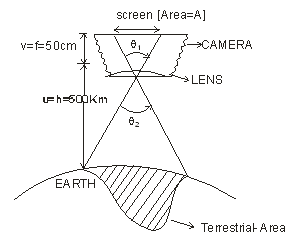Question 14: Letters A, B, C and D are written on a cardboard as shown in the picture.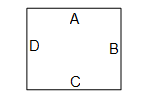The cardboard is kept at a suitable distance behind a transparent empty glass of cylindrical shape. If the glass is now filled with water, one sees an inverted image of the pattern on the cardboard when looking through the glass. Ignoring magnification effects, the image would appear as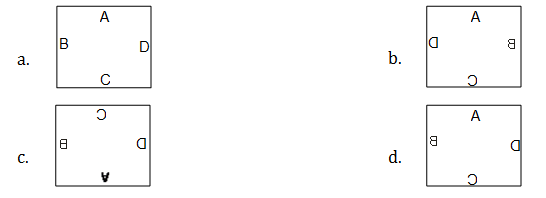Solution:

Given:-

Lens is of cylindrical shape and we know the image formed by cylindrical lens is Erect & Laterally inverted.

Hence option (d) is correct Answer

Question 15: If a ball is thrown at a velocity of 45 m/s in vertical upward direction, then what would be the velocity profile as function of height? Assume g = 10 m/s2.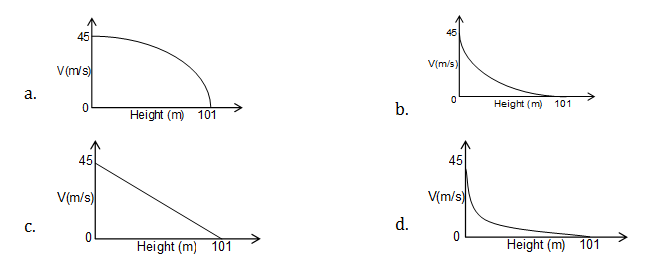Solution:

From Newton’s Third equation of motion

V2 = u2 + 2as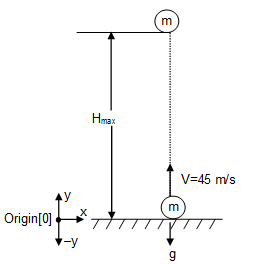Here:- a = – g

At ,S = Hmax = h

V = 0

$h=\frac{V^{2}}{2g}=\frac{(45)^{2}}{2\times 10}$

h = 101.25 m = 101 m

As h = V2/2g

at s = 0 ; V = 45m/s

at s = h ; V = 0

$h\propto V^{2}$ – Parabolic- Variation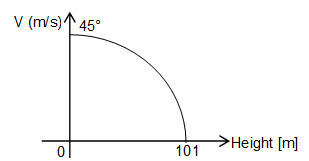Hence; option (A) is correct

Question 16: A coffee maker makes coffee by passing steam through a mixture of coffee powder, milk and water. If the steam is mixed at the rate of 50 g per minute in a mug containing 500 g of mixture, then it takes about t0 seconds to make coffee at 70°C when the initial temperature of the mixture is 25°C. The value of t0 is close to (ratio of latent heat of evaporation to specific heat of water is 540°C and specific heat of the mixture can be taken to be the same as that of water)

1. a. 30
2. b. 45
3. c. 60
4. d. 90

Solution:

1. From principle of calorimetry:

Heat provided by steam = Heat gain by mixture

For Steam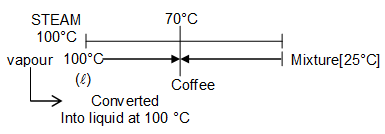Qsteam = Qmixture

MsL + MsS Δ θs = Mm SΔ θm

From the definition of Latent Heat = MsL

From the definition of Specific Heat = MsSΔ θs

From the definition of Specific Heat = Mm SΔ θm

$\frac{\Delta M_{s}}{\Delta t}[L+S\Delta \theta _{s}]=M_{m}\frac{S\Delta \theta _{m}}{\Delta t}$

$\frac{\Delta \theta _{m}}{\Delta t}=\frac{\Delta M_{s}/\Delta t}{M_{m}}\left [ \frac{L}{S}+\Delta \theta _{s} \right ]$

Substituting the values

$\frac{45}{\Delta t}=\frac{50}{500}[540+30]$

$\Delta t=\frac{45}{57}\times 60 second$

$\Delta t=45 second$(approx)

Question 17: A person in front of a mountain is beating a drum at the rate of 40 per minute and hears no distinct echo. If the person moves 90 m closer to the mountain, he has to beat the drum at 60 per minute to not hear any distinct echo. The speed of sound is

1. a. 320 ms–1
2. b. 340 ms–1
3. c. 360 ms–1
4. d. 380 ms–1

Solution:

For not hearing Echo, time interval between the beats of drum must be equal to the time of Echo.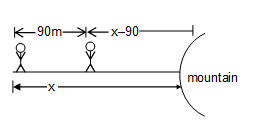t1= 2x/V

[f1 =1/t1]

$\frac{60}{40}=t_{1}=\frac{2x}{V}$

$\frac{3}{2}=\frac{2x}{V}$------------------(1)

Now,

f2 = 60 per minute

f2 = 2[x-90]/V

$\frac{60}{60}=\frac{2x}{V}-\frac{180}{V}$ [Substituting the value of 2x/V from equation (1)]

$1=\frac{3}{2}-\frac{180}{V}$

$\frac{180}{V}=\frac{1}{2}$

V= 360 m/s

Question 18: A glass beaker is filled with water up to 5 cm. It is kept on top of a 2 cm thick glass slab. When a coin at the bottom of the glass slab is viewed at the normal incidence from above the beaker, it’s apparent depth from the water surface is d cm. Value of d is close to (the refractive indices of water and glass are 1.33 and 1.50, respectively)

1. a. 2.5
2. b. 5.1
3. c. 3.7
4. d. 6.0

Solution: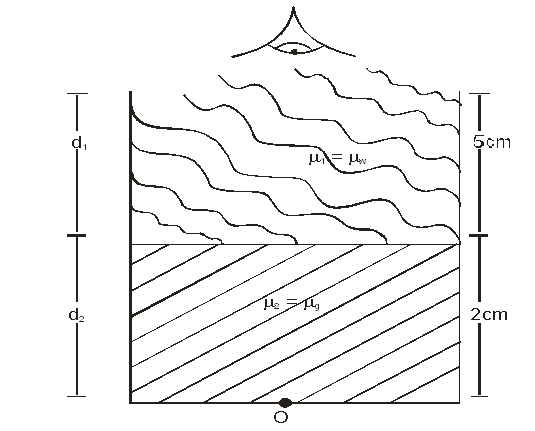$Apparent-depth[d]=\frac{d_{1}}{\mu _{1}}+\frac{d_{2}}{\mu _{2}}$

$Apparent-depth[d]=\frac{d_{1}}{\mu _{w}}+\frac{d_{2}}{\mu _{g}}$

Substituting the values of µw and µg

= $\frac{5}{\left [ \frac{4}{3} \right ]}+\frac{2}{\left [ \frac{3}{2} \right ]}$

= $\frac{15}{4}+\frac{4}{3}$

d=5.1cm

Question 19: A proton of mass m and charge e is projected from a very larger distance towards an α particle with velocity v. Initially, α particle is at rest, but it is free to move. If gravity is neglected, then the minimum separation along the straight line of their motion will be

1. a. e2/4 π ε0mv2
2. b. 5e2/4 π ε0mv2
3. c. 2e2/4 π ε0mv2
4. d. 4e2/4 π ε0mv2

Solution: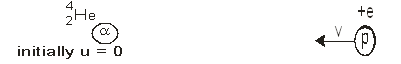From conservation of linear momentum [As ∑F = 0]

Pinitial = Pfinal

mv = [m + 4 m] V’

v = 5V’

V’ = v/5

Now from conservation of mechanical energy:-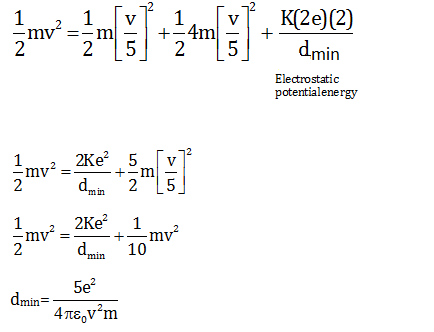Method II

Applying conservation of mechanical Energy with respect to centre of mass frame:-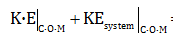= potential energy at minimum separation dmin

$\frac{1}{2}\mu v_{rel}^{2}=\frac{K(2e)(e)}{d_{min}}$

Where, 2e=charge of α particle, e=charge of proton

And K=Coulomb's constant=1/4πε0

μ = Reduced Mass = m1m2/m1+m2

Where, m1= mass of α-particle and m2= mass of proton

$\mu =\frac{(4m)(m)}{(4m+m)}=\frac{4m}{5}$

$\frac{1}{2}\left [ \frac{4m}{5} \right ]v^{2}=\frac{2Ke^{2}}{d_{min}}$

$d_{min}=\frac{5e^{2}}{4\pi \varepsilon _{0}v^{2}m}$

Question 20: A potential is given by V(x) = k(x + a)2/2 for x < 0 and V(x) = k(x – a)2/2 for x > 0. The schematic variation of oscillation period (T) for a particle performing periodic motion in this potential as a function of its energy E is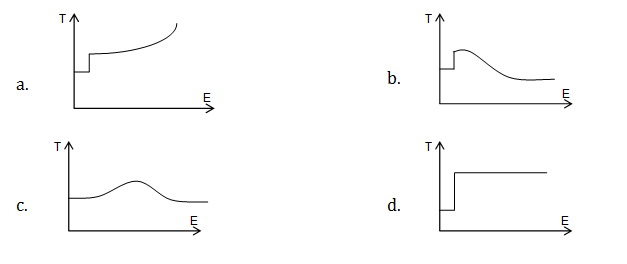Solution: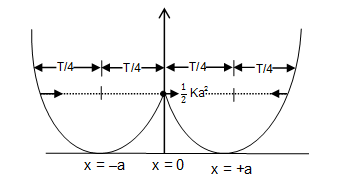For small energy; particle oscillates in one well either it will oscillate about x = +a OR x = –a & Time period of this oscillation is T. [Below energy Level (1/2)Ka2 ]

When this particle gain energy equal to the [(1/2)Ka2 ] it will oscillate in both well so total time period now become 2T so there is a sudden jump in time period.

Now as you increase energy more than [(1/2)Ka2 ] then: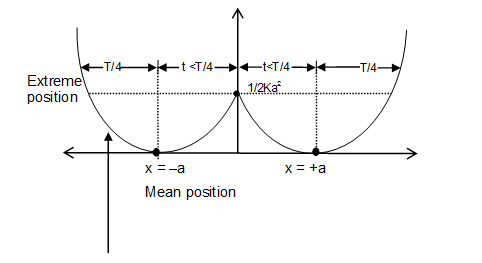[This curve indicates that it takes less than T/4 time period from x = – a to x = 0]

Hence time period starts decreasing from 2T. Hence option B is correct.

Now for high energy we can conclude that curve gets symmetrical and time period again asymptotically approaches T.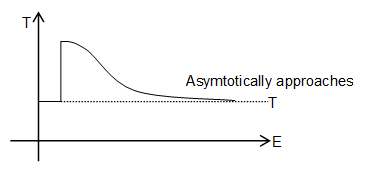### KVPY-SA 2018 Physics Paper with Solutions